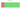# ZWL\$

## Zimbabwean Dollar

### Zimbabwean dollar (2009)

#### how has the value of the currency changed in the last year?## USD 1 = ZWL 322

How much is10 US dollars worth inZimbabwean dollars (2009)?
At the current exchange rate, 10 US dollars is worth 3,220 Zimbabwean dollars (2009)
How much is50 US dollars worth inZimbabwean dollars (2009)?
At the current exchange rate, 50 US dollars is worth 16,100 Zimbabwean dollars (2009)
How much is100 US dollars worth inZimbabwean dollars (2009)?
At the current exchange rate, 100 US dollars is worth 32,200 Zimbabwean dollars (2009)
How much is500 US dollars worth inZimbabwean dollars (2009)?
At the current exchange rate, 500 US dollars is worth 161,000 Zimbabwean dollars (2009)
How much is2,000 US dollars worth inZimbabwean dollars (2009)?
At the current exchange rate, 2,000 US dollars is worth 644,000 Zimbabwean dollars (2009)
How much is10 US dollars worth inZimbabwean dollars (2009)?
At the current exchange rate, 10 US dollars is worth 3,220 Zimbabwean dollars (2009)
How much is50 US dollars worth inZimbabwean dollars (2009)?
At the current exchange rate, 50 US dollars is worth 16,100 Zimbabwean dollars (2009)
How much is100 US dollars worth inZimbabwean dollars (2009)?
At the current exchange rate, 100 US dollars is worth 32,200 Zimbabwean dollars (2009)
How much is500 US dollars worth inZimbabwean dollars (2009)?
At the current exchange rate, 500 US dollars is worth 161,000 Zimbabwean dollars (2009)
How much is2,000 US dollars worth inZimbabwean dollars (2009)?
At the current exchange rate, 2,000 US dollars is worth 644,000 Zimbabwean dollars (2009)